﻿ 基于切比雪夫多项式预测我国居民杠杆率变化及对我国房地产业发展建议

# 基于切比雪夫多项式预测我国居民杠杆率变化及对我国房地产业发展建议Prediction of Changes in China’s Resident Leverage Based on Chebyshev Polynomials and Suggestions for the Development of China’s Real Estate Industry

Abstract: For a long time, everyone has formed a deep-rooted impression: Compared with developed coun-tries, Chinese residents have lower leverage and less repayment pressure. Therefore, there is a lot of room for residents to increase leverage, and there is still room for housing price bubble growth . This article will analyze China’s residents’ leverage ratio and predict future residents’ leverage ratio through Chebyshev polynomials. At the same time, we will compare our residents’ leverage ratio with other countries to explore the true situation of our residents’ leverage ratio and the real estate industry suggestions for development.

1. 中国的房地产业发展历程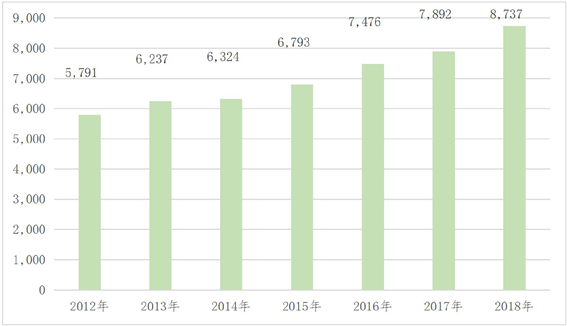Figure 1. Average selling price of houses (yuan/square meter)

2. 居民贷款买房现状

2.1. 住户部门杠杆率处于国际平均水平，但增速仍高于其他主要经济体

2018年末，我国住户部门杠杆率为60.4%。从国际同比看，我国住户部门杠杆率与国际平均水平一致，低于发达经济体平均水平，但在新兴市场经济体中处于较高水平(见图2)。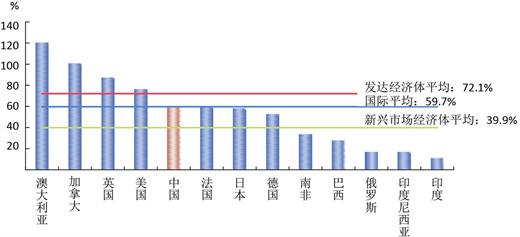Figure 2. Household sector leverage ratio in some economies at the end of 2018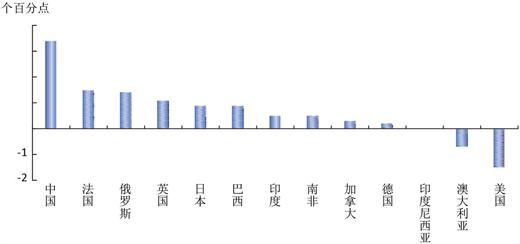Figure 3. Changes in the household sector leverage ratio in some economies in 2018

2.2. 债务收入比保持高速增长，低收入家庭债务负担较重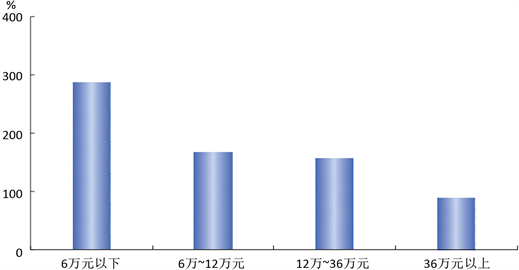Figure 4. Debt-income ratio of households with different income levels

2.3. 东南沿海地区住户部门债务风险相对较高

3. 用切比雪夫多项式拟合预测我国居民杠杆率变化

3.1. 数据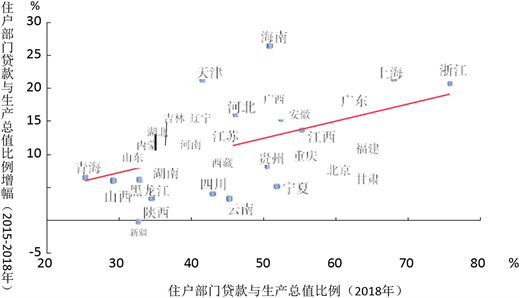Figure 5. Household sector loan to GDP ratio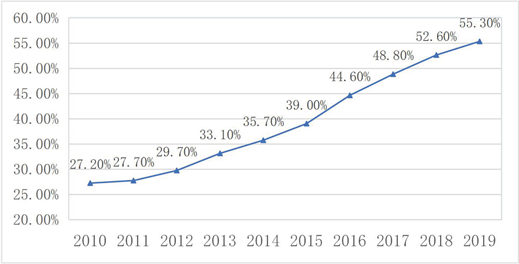Figure 6. Chinese residents’ pole rate

3.2. 拟合原理

${T}_{0}\left(x\right)=1$ (1)

${T}_{1}\left(x\right)=x$ (2)

${T}_{n+1}\left(x\right)=2x{T}_{n}\left(x\right)-{T}_{n-1}\left(x\right)$ (3)

$p\left(x\right)={a}_{0}{T}_{0}\left(x\right)+{a}_{1}{T}_{1}\left(x\right)+{a}_{2}{T}_{2}\left(x\right)+{a}_{3}{T}_{3}\left(x\right)\cdots$ (4)Figure 7. Chebyshev polynomial image

$\left(T-1980\right)/\left(2030-1980\right)=\left(x+1\right)/2$ (5)

$x=2\ast \left(T-a\right)/\left(b-a\right)-1,\text{\hspace{0.17em}}T=\left(x+1\right)\ast \left(b-a\right)/2+a$ (7)

3.3. 拟合结果

3.4. 拟合的局限和不足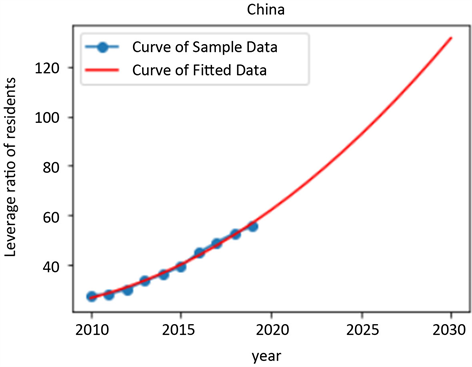Figure 8. Chebyshev polynomial prediction of residents’ leverage in China

4. 我国居民杆杆率变化原因分析

4.1. 商品房价格居高不下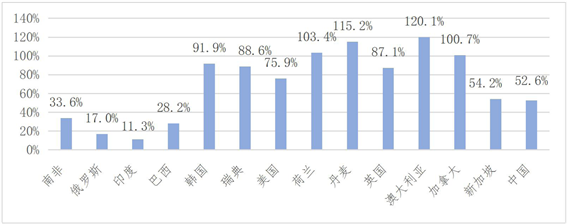Figure 9. Residents leverage ratio in 2018

4.2. 新时代的消费理念

2014年末以来，为提振经济、推动房地产业去库存，国家不断推出利好政策，刺激购房需求陆续释放，购房贷款迅速上涨 。

2018年《中国养老前景调查报告》指出，在受访的18到34岁之间的年轻人中，平均每人每月储蓄只有1339元。现在的年轻人为什么存钱做的都普遍不好呢？支付宝的花呗、京东的白条以及信用卡，还有更多此类的超前消费方式正在暗示着年轻人不停地买买买，随后等到还款日却只好眼看着手中的银行卡里本就不多的余额瞬间掏空，因此以后只能靠信用卡生活。并且据统计，2018年的8月份末期，我国信用卡贷款年均增长近32.9%，消费性贷款规模达到了31.5万亿元，到2018年一直保持20%以上的年均增速，如图10所示。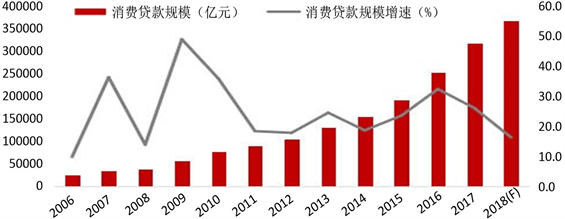Figure 10. China’s consumer scale loan scale and growth

4.3. 住房过度消费

5. 建议与总结

5.1. 财政税收

5.1.1. 差别化的税收调控政策

5.1.2. 施行房地产保有税

5.1.3. 对投资性住房转让课以重税

5.2. 房源供给侧改革

 姜超. 中国居民房贷杠杆真的不低了[J]. 宏观热评, 2016(37): 16-17.

 庄涵. 我国房地产行业发展历程[J]. 广西质量监督导报, 2019(3): 46-47.

 中国金融稳定报告[EB/OL]. https://baike.so.com/doc/26527815-27791195.html

 顾乐民. 预测型切比雪夫多项式[J]. 计算机工程与应用, 2012, 48(7): 34-38.

 左小蕾. 房地产价格为何居高不下? [J]. 上海国资, 2013(3): 16.

 王宏涛, 李岚, 张露. 住房贷款对我国居民家庭消费的影响研究[EB/OL]. 金融发展研究, 1-10.
https://doi.org/10.19647/j.cnki.37-1462/f.2020.03.004, 2020-03-29.

 孙丹, 李宏瑾. 居民杠杆率、房地产信贷与房价泡沫风险[J]. 金融发展评论, 2017(1): 30-41.

 住房过度消费不可取[J]. 领导决策信息, 2000(31): 21.

Top